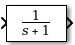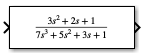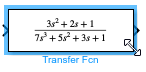# Transfer Fcn

Model linear system as transfer function

•Libraries:

## Description

The Transfer Fcn block models a linear system by a transfer function of the Laplace-domain variable `s`. The block can model single-input single-output (SISO) and single-input multiple-output (SIMO) systems.

### Conditions for Using This Block

The Transfer Fcn block assumes the following conditions:

• The transfer function has the form

`$H\left(s\right)=\frac{y\left(s\right)}{u\left(s\right)}=\frac{num\left(s\right)}{den\left(s\right)}=\frac{num\left(1\right){s}^{nn-1}+num\left(2\right){s}^{nn-2}+\dots +num\left(nn\right)}{den\left(1\right){s}^{nd-1}+den\left(2\right){s}^{nd-2}+\dots +den\left(nd\right)},$`

where u and y are the system input and outputs, respectively, nn and nd are the number of numerator and denominator coefficients, respectively. num(s) and den(s) contain the coefficients of the numerator and denominator in descending powers of s.

• The order of the denominator must be greater than or equal to the order of the numerator.

• For a multiple-output system, all transfer functions have the same denominator and all numerators have the same order.

### Modeling a Single-Output System

For a single-output system, the input and output of the block are scalar time-domain signals. To model this system:

1. Enter a vector for the numerator coefficients of the transfer function in the Numerator coefficients field.

2. Enter a vector for the denominator coefficients of the transfer function in the Denominator coefficients field.

### Modeling a Multiple-Output System

For a multiple-output system, the block input is a scalar and the output is a vector, where each element is an output of the system. To model this system:

1. Enter a matrix in the Numerator coefficients field.

Each row of this matrix contains the numerator coefficients of a transfer function that determines one of the block outputs.

2. Enter a vector of the denominator coefficients common to all transfer functions of the system in the Denominator coefficients field.

### Specifying Initial Conditions

A transfer function describes the relationship between input and output in Laplace (frequency) domain. Specifically, it is defined as the Laplace transform of the response (output) of a system with zero initial conditions to an impulse input.

Operations like multiplication and division of transfer functions rely on zero initial state. For example, you can decompose a single complicated transfer function into a series of simpler transfer functions. Apply them sequentially to get a response equivalent to that of the original transfer function. This will not be correct if one of the transfer functions assumes a non-zero initial state. Furthermore, a transfer function has infinitely many time domain realizations, most of whose states do not have any physical meaning.

For these reasons, Simulink® presets the initial conditions of the Transfer Fcn block to zero. To specify initial conditions for a given transfer function, convert the transfer function to its controllable, canonical state-space realization using `tf2ss` . Then, use the State-Space block. The `tf2ss` utility provides the `A`, `B`, `C`, and `D` matrices for the system.

For more information, type `help tf2ss` or see the Control System Toolbox™ documentation.

### Transfer Function Display on the Block

The Transfer Fcn block displays the transfer function depending on how you specify the numerator and denominator parameters.

• If you specify each parameter as an expression or a vector, the block shows the transfer function using the specified coefficients for the powers of s. If you specify a variable in parentheses, the block evaluates the variable.

For example, if you specify the Numerator coefficients parameter value as `[3 2 1]` and the Denominator coefficients parameter value as `(den)`, where `den` is a workspace variable with a value of `[7 5 3 1]`, the block displays the equation using the specified values.Tip

When the block size is too small to accommodate the full numerator or denominator, the block icon displays the numerator as `num(s)` and the denominator as `den(s)`.If you want the block to show the equation for the transfer function it implements, resize the block by dragging a corner.• If you specify each parameter as a variable, the block shows the variable name followed by `(s)`.

For example, if you specify the Numerator coefficients parameter as `num` and the Denominator coefficients parameter as `den`, the block icon shows the numerator of the transfer function as `num(s)` and the denominator as `den(s)`## Ports

### Input

expand all

Input signal, specified as a scalar with data type `double`.

This port has direct feedthrough only when the numerator of the transfer function is `0`.

Data Types: `double`

### Output

expand all

Output signal, provided as a scalar or vector with data type `double`.

• For a single-output system, the input and output of the block are scalar time-domain signals.

• For a multiple-output system, the input is a scalar, and the output is a vector, where each element is an output of the system.

Data Types: `double`

## Parameters

expand all

Define the numerator coefficients of the transfer function.

• For a single-output system, enter a vector for the numerator coefficients of the transfer function.

• For a multiple-output system, enter a matrix. Each row of this matrix contains the numerator coefficients of a transfer function that determines one of the block outputs.

#### Programmatic Use

 Block Parameter: `Numerator` Type: character vector, string Values: vector | matrix Default: `''`

Define the row vector of denominator coefficients.

• For a single-output system, enter a vector for the denominator coefficients of the transfer function.

• For a multiple-output system, enter a vector containing the denominator coefficients common to all transfer functions of the system.

#### Programmatic Use

 Block Parameter: `Denominator` Type: character vector | string Values: vector Default: `'[1 1]'`

Tunability level of the numerator and denominator coefficients for Accelerated simulation modes and deployed simulations using Simulink Compiler™. Set this parameter to `Auto` to allow Simulink to choose the appropriate level of parameter tunability.

Set this parameter to `Optimized` to generate a representation of numerator and denominator coefficients in generated code for accelerated and deployed simulations that is optimized for better simulation performance.

Set this parameter to `Unconstrained` to generate a fully tunable (between simulations) representation of the numerator and denominator coefficients in the generated code for accelerated and deployed simulations. To let Simulink determine the appropriate tunability level, select `Auto` .

#### Programmatic Use

 Block Parameter: `ParameterTunability` Type: character vector, string Values: `'Auto'` | `'Optimized'` | `'Unconstrained'` Default: `'Auto'`

Variable-step solvers use absolute and relative tolerances when choosing the step size to determine whether the error in state calculations is acceptable.

To inherit the absolute tolerance from the Absolute tolerance configuration parameter, specify this parameter value as `auto` or `-1`.

To specify an absolute tolerance for this block that overrides the value specified for the Absolute tolerance configuration parameter:

• Enter a real, positive scalar value to use to compute all block states.

• Enter a real vector with dimensions that match the dimensions of the continuous states for the block.

#### Programmatic Use

 Block Parameter: ` AbsoluteTolerance` Type: string | character vector Values: `'auto'` | `'-1'` | positive, real scalar | vector of positive, real scalars Default: `'auto'`

Use this parameter to optionally assign names to the states of this block. The names you assign apply only to the states of this block.

• To use default state names, leave this field blank (`''`).

• To assign a single name to a single state, enter the name between quotes. For example, to name a single state `position`, enter `"position"`.

• To assign names to multiple states, specify this parameter value as a cell array of character vectors. Each name in the cell array must be unique. For example, to assign the names `a`, `b`, and `c`, enter `{'a','b','c'}`.

• To specify the names using a MATLAB variable, enter the name of the variable without quotes. For example, to use the variable `names` to specify the state names, enter `names`.

You can specify a number of names that is less than the number of states in the block. In this case, the state names are used for multiple states, and the number of states must divide evenly into the number of state names. For example, when you specify two names for a block that has four states, the first name is used for the first two states, and the second name is used for the last two states.

#### Programmatic Use

 Block Parameter: `ContinuousStateAttributes` Type: string | character vector Values: `' '` | user-defined Default: `' '`

## Block Characteristics

 Data Types `double` Direct Feedthrough `noa` Multidimensional Signals `no` Variable-Size Signals `no` Zero-Crossing Detection `no` a Direct feedthrough characteristics for this block depend on block parameter values.

## Version History

Introduced before R2006a